Essentials of Geometry
Reasons & Proofs
Parallel & Perpendicular Lines
Triangles
Circles
Angles/
Transformations
100

The points are colinear with line m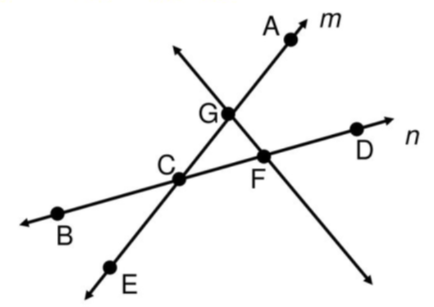What are points A, G, C, and E?

100

The first three members of a sequence are shown. The fourth member of the sequence is ______ total squares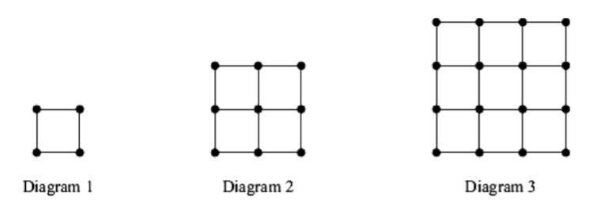What is 16?

100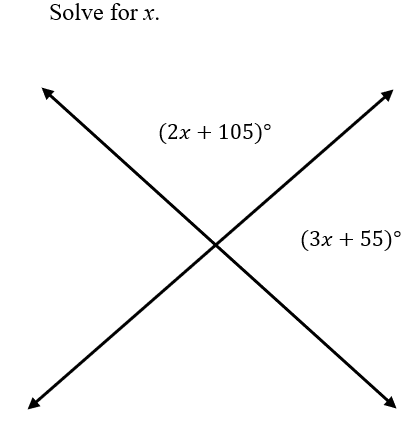What is

x=4?

100

If line segment DE is the midsegment of triangle ABC, The value of x is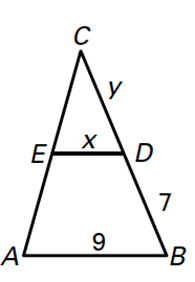What is

x=4.5?

100

The term best describes line segment IH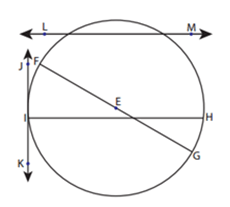What is a chord?

100

The image of Z(- 4, - 3) using the translation (x, y) to (x + 3, y + 4)

What is Z' (-1, 1)?

200

Find the midpoint of the segment with endpoints (5, 4) and (3, -4)

What is (4, 0)?

200

Rewrite the statement in if-then form.

All 90-degree angles are right angles.

What is "If the measure of an angel is 90 degrees, then it is a right angle?"

200

In the diagram, transversal t cuts parallel lines m and n. The measure of angle JQL is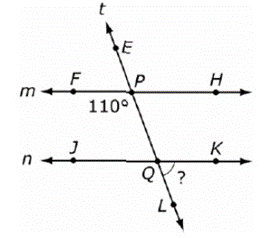What is

110°?

200

In the diagram, which method can be used to prove that these two triangles are congruent? (SSS, SAS, ASA, or AAS)What is SAS?

200

Find the measure of the arc or central angel indicated. Assume that lines which appear to be diameters are actual diameters.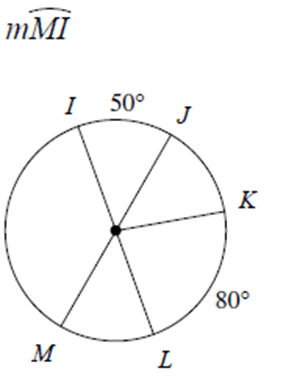What is

130° ?

200

The image of V(- 2, - 4) using the translation (x, y) to (x + 3, y + 4)

What is V' (1, 0)?

300

Find the midpoint of the segment with endpoints (7, 6) and (3, 5)

What is (5, 5.5)?

300

Fill in the blanks for a two-column proof of the theorem: #28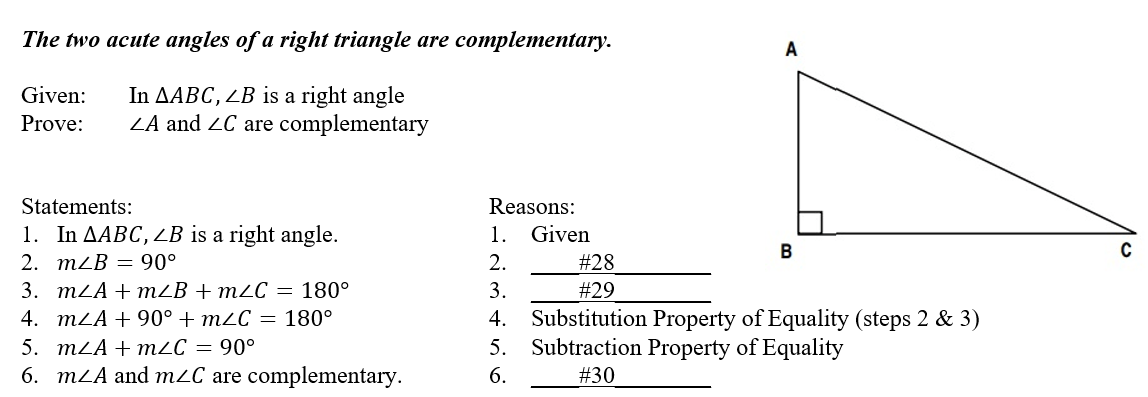What is the definition of a right angles?

300

In the diagram, line t intersects lines m and n. The angel must be supplementary to angle 5 for lines m and n to be parallel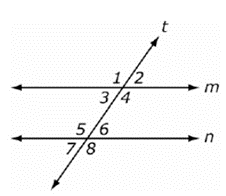What is

∠ 3?

300

List the angles of the triangle from least to greatest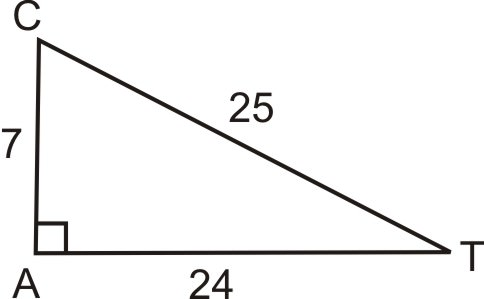What is angle T, angle C, and angle A?

300

Find the indicated measureWhat is

m ∠1=105°?

300

In the diagram, the measure of angle BCE is 144 degrees. Find the measure of xWhat is

x=20?

400

Find the distance between the points (2, 6) and (–4, –3)

What is

3 √13?

400

Fill in the blanks for a two-column proof of the theorem: #29What is Triangle Sum Theorem?

400

In the diagram to the right, transversal t cuts parallel lines m and n. The angel that is congruent to angle 7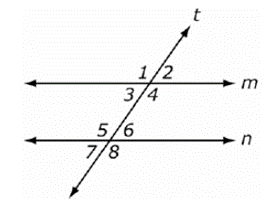What is

∠ 2?

400

The value of  x to the nearest tenth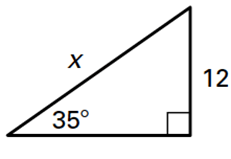What is

x=20.9?

400

Find the indicated measureWhat is

m(XY) ̂=160°?

400

In the diagram, the measure of angle BCE is 144 degrees. Find the measure of angle ECDWhat is

m ∠ECD=72°?

500

Find the distance between the points (3, 4) and (2, 2)

What is

√5?

500

Fill in the blanks for a two-column proof of the theorem: #30What is the definition of complementary angles?

500

In the diagram to the right, line t intersects lines m and n. For what value of x are lines m and n parallel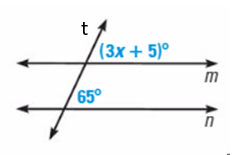What is

x=20?

500

The value of b to the nearest tenth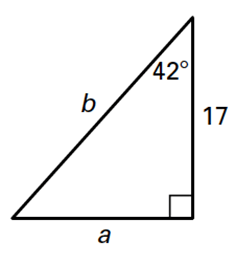What is

b=22.9?

500

Find the indicated measure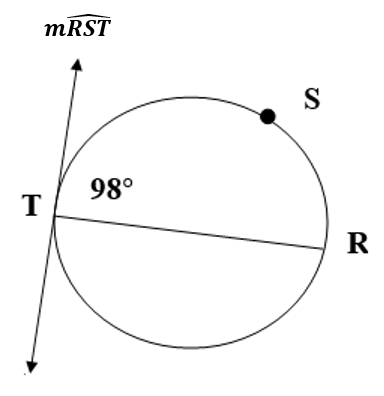What is

m(RST) ̂=196°?

500

In the diagram, the measure of angle BCE is 144 degrees. Find the measure of angle BCDWhat is

m ∠BCD=72°?

Click to zoom